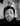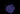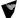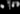Navigate back to the homepage

# Abstract Blob Shader with ParticlesRick
August 8th, 2023 · 4 min readThere are two things going on in this codeSandbox:

1. Inner sphere blob which has noise applied to it
2. A points mesh with the outer smokey particles

## Inner sphere blob which has noise applied to it

So this article gave me an idea to apply turbulence to the surface of a sphere and this is what gives it the super nova type look - that and noise + 2 mixed colors.

```.css-1chxjt6{position:absolute;right:22px;top:24px;padding:8px 12px 7px;border-radius:5px;color:#6f7177;-webkit-transition:background 0.3s ease;transition:background 0.3s ease;}.css-1chxjt6:hover{background:rgba(255,255,255,0.07);}.css-1chxjt6[data-a11y="true"]:focus::after{content:"";position:absolute;left:-2%;top:-2%;width:104%;height:104%;border:2px solid var(--theme-ui-colors-accent,#6166DC);border-radius:5px;background:rgba(255,255,255,0.01);}@media (max-width:45.9375em){.css-1chxjt6{display:none;}}1const SphereShell = () => {2  const matRef = useRef();3  const sphereShellRef = useRef();4
5  const customShader = {6    uniforms: {7      time: {8        value: 09      }10    }11  };12
27        varying vec2 vUv;28        varying vec3 vPosition;29        varying float vNoise; 30
31
32        \${cnoise}33
34        float turbulence( vec3 p ) {35
36          float w = 100.0;37          float t = -.5;38
39          for (float f = 1.0 ; f <= 10.0 ; f++ ){40            float power = pow( 2.0, f );41            t += abs( cnoise( vec4( power * p, 1.0 ) ) / power );42          }43
44          return t;45
46        }47
56
57        vUv = uv;58
59        // get a turbulent 3d noise using the normal, normal to high freq60        float noise = 10.0 *  -.10 * turbulence( .5 * normal + time * 0.25);61        // get a 3d noise using the position, low frequency62        float b = .30 * cnoise( vec4(0.05 * position, 1.0) );63        // compose both noises64        float displacement = - 1. * noise + b;65      66        // move the position along the normal and transform it67        vec3 newPosition = position + normal * displacement;68        transformed = newPosition;69        vPosition = newPosition;70        vNoise = noise;71      `72      );73
79        uniform float time;80        varying float vNoise;81        varying vec3 vPosition;82
91        float scale = 10.0;92        float radius = mod(time * 4.0, 20.0);93
94        float contrast = 20.2;95        vec3 blue = vec3(0.1,0.3,2.0) * vNoise * 0.1;96        vec3 lesserBlue = vec3(0.05, 0.15,1.0)* vNoise * 0.03;97
98        float noise = vNoise + 0.3;99
100        diffuseColor = vec4(101          mix(lesserBlue, blue , noise) * contrast + 0.2,102          mix(103           0.0,104            1.0, 105            1.0 - (radius / 20.0)  106          )107        ) ;108      `109      );110
116  useFrame(({ clock, gl }) => {117    if (matRef.current) {118      const shader = matRef.current.userData.shader;119
120      if (shader) {121        shader.uniforms.time = {122          value: clock.elapsedTime123        };124      }125    }126
127    if (sphereShellRef.current) {128      const scale = (clock.elapsedTime * 4.0) % 20.0;129      sphereShellRef.current.scale.set(scale, scale, scale);130    }131  });132
133  return (134    <group>135      <ambientLight />136      <mesh ref={sphereShellRef}>137        <sphereGeometry args={[1.0, 100, 100]} />138        <meshStandardMaterial139          ref={matRef}140          onBeforeCompile={OBC}141          transparent142          side={THREE.DoubleSide}143        />144      </mesh>145    </group>146  );147};```

This is an example of the official way to adapt builtin materials. Why is this a good way to do it?

Well… unless your a physicist or a computer scientist which can understand complicated equations and convert them into code (which is a hard and rare skill to have), then this is definitely the way to go.

It injects code before the shader gets compiled and therefore allows you to inject snippets into positions of the built in shader files or chunks.

The .replace builtin javascript string method, allows us to select a shader chunk name or piece of code we know is in the built in shader. Then replace it with itself and the new code.

Unfortunately this does require some knowledge of what shader terms mean and quite a bit of playing around. For example instead of setting gl_FragColor it could be diffuseColor or transformed in the vertex shader instead of setting gl_Position.

Because we don’t want to set the color or vertex position in the wrong place as this defeats the point of merging our code in seamlessly and taking advantage of all the light calculations. Its all very well creating a abstract shader but if you want nice colors and lighting you will need to implement it yourself or use this onBeforeCompile.

## Update uniforms using onBeforeCompile

This took me a while to get right!

In the onBeforeCompile we use the materials userData object and we store a reference to the shader in the onBeforeCompile callback.

We obtain the shader parameter from the first param of onBeforeCompile callback and then set it as a property on the userData object on the material. Important to note… this now gives us a reference to the shader which we can now access the uniforms of the shader and update as you would in the normal way in the useFrame R3F hook.

Sounds more complicated than it actually is and is one of the official ways to access and update uniforms using onBeforeCompile.

```1// define the mesh with OBC2<mesh ref={sphereShellRef}>3    <sphereGeometry args={[1.0, 100, 100]} />4    <meshStandardMaterial5        ref={matRef}6        onBeforeCompile={OBC}7        transparent8        side={THREE.DoubleSide}9    />10</mesh>11
12// set the shader object in OBC13// callback to material useData object14
22
25

This is where we apply turbulence and pass some varying’s to the fragment shader to create a nice colorful effect.

```1shader.vertexShader = shader.vertexShader.replace(2    "#include <clipping_planes_pars_vertex>",3    /* glsl */ `4    5    #include <clipping_planes_pars_vertex>6    uniform float time;7
8    varying vec2 vUv;9    varying vec3 vPosition;10    varying float vNoise; 11
12
13    \${cnoise}14
15    float turbulence( vec3 p ) {16
17        float w = 100.0;18        float t = -.5;19
20        for (float f = 1.0 ; f <= 10.0 ; f++ ){21        float power = pow( 2.0, f );22        t += abs( cnoise( vec4( power * p, 1.0 ) ) / power );23        }24
25        return t;26
27    }28
37
38    vUv = uv;39
40    // get a turbulent 3d noise using the normal, normal to high freq41    float noise = 10.0 *  -.10 * turbulence( .5 * normal + time * 0.25);42    // get a 3d noise using the position, low frequency43    float b = .30 * cnoise( vec4(0.05 * position, 1.0) );44    // compose both noises45    float displacement = - 1. * noise + b;46    47    // move the position along the normal and transform it48    vec3 newPosition = position + normal * displacement;49    transformed = newPosition;50    vPosition = newPosition;51    vNoise = noise;52    `53);```

So if we have a singular vertex or fragment shader, why do we have to do the replace twice?

The first replace is for any functions, uniforms, varyings, defines or pragmas with glslify. And the second one is for any code going into the body of the main function in the shaders.

`#include <clipping_planes_pars_vertex>` - is at the end of all the uniforms and is perfect place for putting all the top elements in.

`#include <begin_vertex>` - is at a point in the main function where we can access the transformed vec3 which is before any matrix multiplications (as far as I know or can tell)

So we are using the turbulence function and apply noise over time we want a quite large noise scale as we don’t want it too wavy like clouds. Then set this to the transformed vec3, which will get used in all the other shader chunks below it - `#include <begin_vertex>`.

The fragment shader is where we add all the color and modify the opacity with noise.

```1shader.fragmentShader = shader.fragmentShader.replace(2"#include <clipping_planes_pars_fragment>",3`4    #include <clipping_planes_pars_fragment>5
6    uniform float time;7    varying float vNoise;8    varying vec3 vPosition;9
18    float radius = mod(time * 4.0, 20.0);19
20    float contrast = 20.2;21    vec3 blue = vec3(0.1,0.3,2.0) * vNoise * 0.1;22    vec3 lesserBlue = vec3(0.05, 0.15,1.0)* vNoise * 0.03;23
24    float noise = vNoise + 0.3;25
26    diffuseColor = vec4(27        mix(lesserBlue, blue , noise) * contrast + 0.2,28        mix(29        0.0,30        1.0, 31        1.0 - (radius / 20.0)  32        )33    );34`35);```

The top replace is as it was for the vertex shader, just the fragment shader with a different string to replace `#include <clipping_planes_pars_fragment>`.

The bottom replace is the interesting part!

So we have an expanding noisy sphere by having an oscillating radius using the builtin `mod()` glsl math function, noting the 20.0 float.

We want to increase the contrast i.e. make the bright parts brighter and the dark parts darker. Basically increase the difference of the spectrum of colors.

Have a play and change it.

We have two blue colors and use noise as a multiplier which gives us the nice set of colors to mix. Finally mixing these two colors with the builtin `mix()` glsl function with noise again - meaning lower values of noise will associate with one color and higher values of noise with the other color.

In these circumstances noise is good.

We increase the brightness of the mixed color by adding a 0.2 float to it. This addition will make things lighter all over. Remember 1.0 is white and 0.0 is black.

Below will make it so that the alpha channel gets smaller as you get further away from the origin.

`1mix(2    0.0,3    1.0, 4    1.0 - (radius / 20.0)  5)`

The first param of `mix()` will be more dominent if the third param is closer to 0 i.e. the radius gets bigger ( noting this `mix()` function’s third param is in the range of 0-1). We divide the radius by 20.0, as above we mod’ed the time by 20.0. All this means the greater the radius the lower the alpha channel will be.

And because we set the transparent prop on the material we can have transparent parts of the material. Important to also note that not setting this will make changing the alpha channel in the fragment shader useless.

## A points mesh with the outer smokey particles

Im not going to spend a lot of time on this as I have covered it in another tutorial. This tutorial did soft particles - granted unlit particles like in this codesandbox, as a builtin points material doesn’t have light affecting it without calculating it manually (I read the light calculations are excluded as it has to do with normal calculations, or calculating normals for points rather than meshes is troublesome).

```1shader.vertexShader = shader.vertexShader.replace(2    "#include <clipping_planes_pars_vertex>",3    /* glsl */ `4    5    #include <clipping_planes_pars_vertex>6    uniform float time;7    uniform vec3 mousePos;8
9    varying vec2 vUv;10    varying float noise;11    varying vec3 noisyPos;12    varying vec3 vNormal;13    varying float vDepth;14
15
16
17    \${cnoise}18
19    float turbulence( vec3 p ) {20
21        float w = 100.0;22        float t = -.5;23
24        for (float f = 1.0 ; f <= 10.0 ; f++ ){25        float power = pow( 2.0, f );26        t += abs( cnoise( vec4( power * p, 1.0 ) ) / power );27        }28
29        return t;30
31    }32
33    float sdSphere( vec3 pos, float radius ) {34            return max(length(pos), 0.0) - radius;35    }36
37    float quadraticInOut(float t) {38        float p = 2.0 * t * t;39        return t < 0.5 ? p : -p + (4.0 * t) - 1.0;40    }41
49    float uSpeed = 14.0;50    float uAmplitude = 9.1; 51
52    vUv = uv;53
54    vec3 tempPos = position;55        56    float uCurlFreq = 40.010;57    vec3 sum = vec3(0.0,0.0,0.0);58    sum += cnoise(vec4(tempPos, 1.0) * uCurlFreq + time * 0.9);59    sum += cnoise(vec4(tempPos, 1.0) * uCurlFreq * 0.025) ;60    sum += cnoise(vec4(tempPos, 1.0) * uCurlFreq * 0.05) ;61    sum += cnoise(vec4(tempPos, 1.0) * uCurlFreq * .10) ;62    sum += cnoise(vec4(tempPos, 1.0) * uCurlFreq * .20) ;63    sum /=5.0;64    tempPos += sum;65
66    transformed = tempPos;67
68    // get a turbulent 3d noise using the normal, normal to high freq69    float noise = 10.0 *  -.10 * turbulence( .5 * normal );70    // get a 3d noise using the position, low frequency71    float b = .70 * cnoise( vec4(0.05 * position + vec3( 10.0 ) + time, 1.0) );72    // compose both noises73    float displacement = - 1. * noise + b;74    75    // move the position along the normal and transform it76    vec3 newPosition = position + normal * displacement * 10.0;77
78    noisyPos = newPosition;79    vNormal = normal;80    vDepth = gl_Position.z / gl_Position.w;81
82    `83);84
85shader.vertexShader = shader.vertexShader.replace(86    `gl_PointSize = size;`,87    /* glsl */ `88    float repeatingRadius  = mod(time * 4.0, 20.0);89    // float eased = quadraticInOut(repeatingRadius / 20.0) * time * 3.0;90    float defaultPointSize = 0.01;91    float maxPointSize = 25.1;92    float minRange = 1.9;93    float outerRange = 2.2;94
95    float sdf = sdSphere(noisyPos, repeatingRadius);96
97    bool inside =  sdf < minRange && sdf > -minRange;98    bool outer = sdf < outerRange && sdf > minRange && sdf < -minRange && sdf > -outerRange;99
100    if (inside) {101        gl_PointSize = mix(defaultPointSize, maxPointSize,   repeatingRadius / 20.0);102    } 103
104    else if (outer) {105        gl_PointSize = mix(maxPointSize, defaultPointSize, repeatingRadius / 20.0);106
107    } else {108        gl_PointSize = defaultPointSize;109    }110    111
112    113    `114);```

Very quickly the top replace defines the properties like uniforms and functions etc, the middle replace adds the turbulence which we will utilize in the fragment shader and finally a third replace deals with an increasing gl_PointSize as the SDF checks if the points are in a certain distance from the crest of the wave.

```1shader.fragmentShader = shader.fragmentShader.replace(2    "#include <clipping_planes_pars_fragment>",3    /* glsl */ `4    #include <clipping_planes_pars_fragment>5
6    uniform float time;7    uniform sampler2D smoke;8    varying vec3 noisyPos;9    varying vec3 vNormal;10    varying float vDepth;11
12    float rand(vec2 co, float seed) {13        float a = 12.9898;14        float b = 78.233;15        float c = 43758.5453;16        float dt= dot(co.xy ,vec2(a,b));17        float sn= mod(dt, 3.14);18        return fract(sin(sn + seed) * c);19    }20
21    vec2 rotateUV(vec2 uv, float rotation, vec2 mid) {22        return vec2(23        cos(rotation) * (uv.x - mid.x) + sin(rotation) * (uv.y - mid.y) + mid.x,24        cos(rotation) * (uv.y - mid.y) - sin(rotation) * (uv.x - mid.x) + mid.y25        );26    }27
36    float repeatingRadius  = mod(time * 4.0, 20.0);37
38    // Easing produces 0-1 so have to scale back up39    // float eased = quadraticInOut(repeatingRadius / 20.0) * time * 2.0;40    vec3 colorInside = vec3(0.1,0.3,10.0) * 43.0 * cnoise(vec4(noisyPos, 1.0)) * (abs(length(normalize(noisyPos))));41    vec3 colorOutside = vec3(0.05, 0.15,0.2) * 0.15;42    float minRange = 1.9;43    float outerRange = 2.2;44
45
46    // Soft Particles47
48    vec2 spriteSheetSize = vec2(1280.0, 768.0);   // In px49    vec2 spriteSize = vec2(256, 256.0);        // In px50    float index = 1.0;            // Sprite index in sprite sheet (0-...)51    float w = spriteSheetSize.x;52    float h = spriteSheetSize.y;53
54    // Normalize sprite size (0.0-1.0)55    float dx = spriteSize.x / w;56    float dy = spriteSize.y / h;57
58    // Figure out number of tile cols of sprite sheet59    float cols = w / spriteSize.x;60
61    // From linear index to row/col pair62    float col = mod(index, cols);63    float row = floor(index / cols);64
65    // Finally to UV texture coordinates66    vec2 uv = vec2(dx * gl_PointCoord.x + col * dx, 1.0 - dy - row * dy + dy * gl_PointCoord.y);67
68    float alpha = texture2D(smoke, uv).a;69
70    //If transparent, don't draw71    if (alpha < 0.01) discard;72
73    vec3 outputColor = vec3(0.2,0.1,3.0);74    outputColor.b += 2.0;75
76    gl_FragColor = vec4(outputColor, (1.0 - repeatingRadius / 20.0) * 0.0199 );77`78);```

Everything up to this code section is covered in the previous article.

```1vec3 outputColor = vec3(0.2,0.1,3.0);2outputColor.b += 2.0;3
4gl_FragColor = vec4(outputColor , (1.0 - repeatingRadius / 20.0) * 0.0199 );```

We want the particles textures to have a blue tinge so we increase the blue channel compared to the other red/green channels and then manually increase its blue channel again to emphasize its blue’ness.

`1(1.0 - repeatingRadius / 20.0) * 0.0199`

This code just means that as the radius (range 1.0-20.0) gets bigger the alpha gets smaller and more transparent, and we fine tune this with the multiplier `* 0.0199`.

Subtle is often better than bold floats or multipliers.

## Particles Geometry and BufferAttributes

```1useEffect(() => {2    const n = 40,3        n2 = n / 2; // particles spread in the cube4
5    for (let i = 0; i < numPoints; i++) {6        // positions7
8        const x = Math.random() * n - n2;9        const y = Math.random() * n - n2;10        const z = Math.random() * n - n2;11
12        // positions.push(x, y, z);13
14        positions[i * 3] = x;15        positions[i * 3 + 1] = y;16        positions[i * 3 + 2] = z;17    }18
19    pointsRef.current.geometry.setAttribute(20        "position",21        new THREE.BufferAttribute(positions, 3)22    );23});```

This small bit of code produces a range of points across a distance in 3D space, in this case 40 three.js units ( -20.0 - +20.0), into a Float32Array which is required for setting a buffer attribute. And we initialize the buffer attribute like so:

`1<bufferGeometry args={[radius, 20, 20]}>2    <bufferAttribute3        attachObject={["attributes", "position"]}4        array={new Float32Array(numPoints * 3)}5        itemSize={3}6        onUpdate={(self) => (self.needsUpdate = true)}7    />8</bufferGeometry>`

Until next time!

### More articles from theFrontDev### Realtime Displacement Maps using CanvasTextures in React Three Fiber

A very quick walkthrough for doing realtime displacement of a subdivided plane using displacement maps in Three.js. This has a wide number of applications, for example terrain tools much like in unity, real time growth patterns or some sort of animated displacement. Combined with emission and potential postprocessing you could create some pretty cool projects with this technique.

August 8th, 2023 · 2 min read### Workflow from Shadertoy to React Three Fiber - R3F

This is a workflow from shadertoy to r3f (@react-three/fiber). It involves ping pong textures and using the output texture as the input texture. This pattern is seen a lot in shadertoy as it uses textures and fragment shaders.

August 7th, 2023 · 5 min read